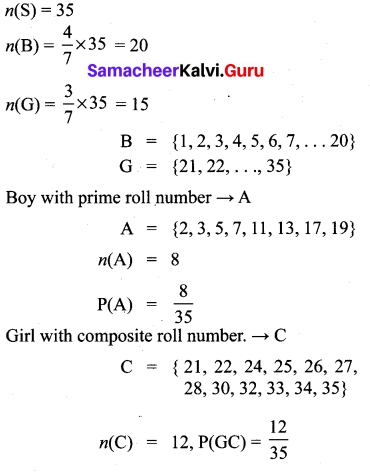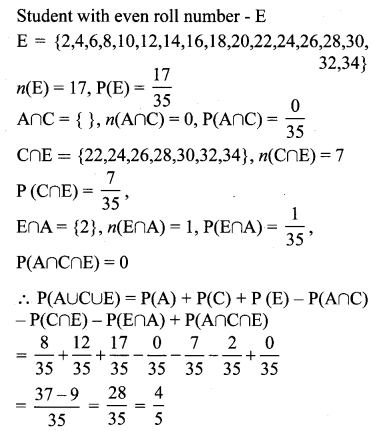# Samacheer Kalvi 10th Maths Solutions Chapter 8 Statistics and Probability Ex 8.4

## Tamilnadu Samacheer Kalvi 10th Maths Solutions Chapter 8 Statistics and Probability Ex 8.4

10th Maths Exercise 8.4 Samacheer Kalvi Question 1.
If P(A) = $$\frac{2}{3}$$, P(B) = $$\frac{2}{5}$$, P(A∪B) = 13 then find P(A∩B).
Solution:
P(A) = $$\frac{2}{3}$$, P(B) = $$\frac{2}{5}$$, P(A∪B) = $$\frac{1}{3}$$
P(A ∩ B) = P(A) + P(B) – P(A∪B)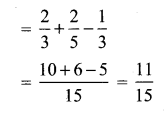Ex 8.4 Class 10 Samacheer Question 2.
A and B are two events such that, P(A) = 0.42, P(B) = 0.48, and P(A ∩ B) = 0.16. Find
(i) P(not A)
(it) P(not B)
(iii) P(A or B)
(i) P(not A) = 1 – P (A) = 1 – 0.42 = 0.58
(ii) P(not B) = 1 – P(B) = 1 – 0.48 = 0.52
(iii) P(A or B) = P(A) + P(B) – P(A ∩ B) = 0.42 + 0.48 – 0.16 = 0.90 – 0.16 = 0.74

10th Maths Exercise 8.4 Question 3.
If A and B are two mutually exclusive events of a random experiment and P(not A) = 0.45, P(A∪B) = 0.65, then find P(B).
Solution:
A and B are two mutually exclusive events of a random experiment.
P(not A) = 0.45,
P(A) = 1 – P(not A)
P(A∪B) = 0.65 = 1 – 0.45 = 0.55
P(A∪B) = P(A) + P(B) = 0.65
0.55 + P(B) = 0.65
P(B) = 0.65 – 0.55
= 0.10

Exercise 8.4 Class 10 Samacheer Question 4.
The probability that atleast one of A and B occur is 0.6. If A and B occur simultaneously with probability 0.2, then find P($$\bar{A}$$) + P($$\bar{B}$$).
Here P (A ∪ B) = 0.6, P (A ∩ B) = 0.2
P (A ∪ B) = P (A) + P (B) – P (A ∩ B)
0.6 = P (A) + P (B) – 0.2
P(A) + P(B) = 0.8
P($$\bar{A}$$) + P($$\bar{B}$$) = 1 – P(A) + 1 – P(B)
= 2 – [P(A) + P(B)]
= 2 – 0.8
= 1.2

10th Maths Statistics And Probability Question 5.
The probability of happening of an event A is 0.5 and that of B is 0.3. If A and B are mutually exclusive events, then find the probability that neither A nor B happen.
Solution:
P(A) = 0.5 Since A and B are mutually inclusive events
P(B) = 0.3 events.
P($$\overline{\mathbf{A}}$$)∪P($$\overline{\mathbf{B}}$$) = 1 – [P(A) + P(B)]
= 1 – [0.5 + 0.3] = 0.2

10th Maths Exercise 8.4 Solution Question 6.
Two dice are rolled once. Find the probability of getting an even number on the first die or a total of face sum 8.
Solution:
Two dice rolled once.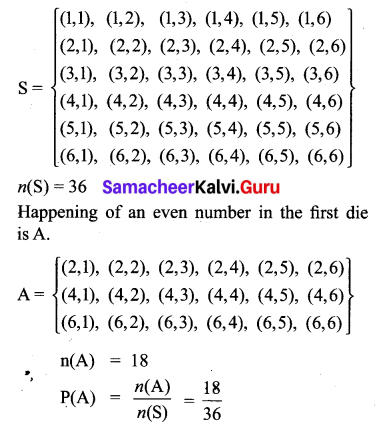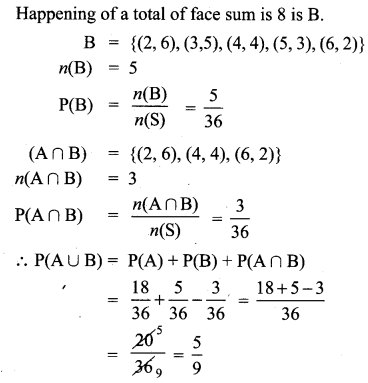Maths Exercise 8.4 Class 10 Question 7.
From a well-shuffled pack of 52 cards, a card is drawn at random. Find the probability of it being either a red king or a black queen.
Solution:
n(S) = 52
No. of Red cards = 26,
Red king cards = 2
No. of Black cards = 26,
Black queen cards = 2
No. of red king cards = n(K) = 2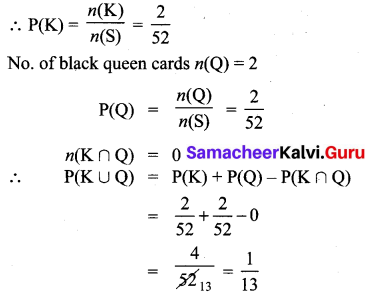∴ The probability of being either a red king or a black queen = $$\frac{1}{13}$$.

Class 10 Maths Ex 8.4 Question 8.
A box contains cards numbered 3, 5, 7, 9,… 35, 37. A card is drawn at random from the box. Find the probability that the drawn card has either multiples of 7 or a prime number.
Solution:
S = {3, 5, 7, 9, 11, 13, 15, 17, 19, 21, 23, 25, 27, 29, 31, 33, 35, 37}
n(S) = 18
Multiplies of seven cards (A) = {7, 21, 35}
= n(A) = 3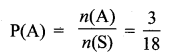Let the prime number cards B
B = {3, 5, 7, 11, 13, 17, 19, 23, 29, 31, 37}
n(B) = 11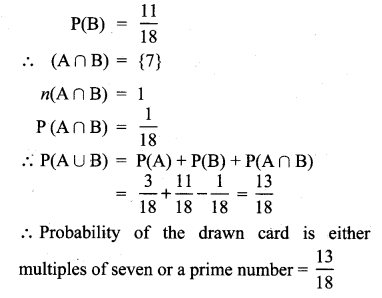10th Class Exercise 8.4 Question 9.
Three unbiased coins are tossed once. Find the probability of getting at most 2 tails or at least 2 heads.
Solution:
When we toss three coins, the sample space S = {HHH, TTT, HTT, THH, HHT, TTH, HTH, THT}
n(S) = 8
Event of getting at most 2 tails be A.
∴ A = { HHH, HTT, THH, HHT, TTH, HTH, THT}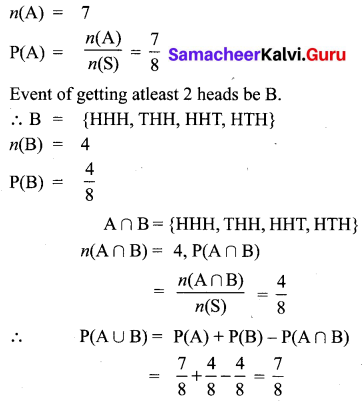Samacheer Kalvi Guru 10th Maths Guide Question 10.
The probability that a person will get an electrification contract is the probability that he will not get plumbing contract is $$\frac{3}{5}$$. The probability of getting at least one contract is $$\frac{5}{8}$$. What is the probability that he will get both?
Solution: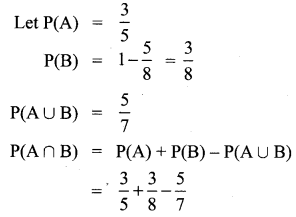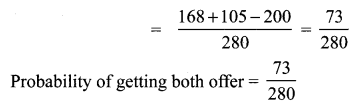10th Class Math Exercise 8.4 Question 11.
In a town of 8000 people, 1300 are over 50 years and 3000 are females. It is known that 30% of the females are over 50 years. What is the probability that a chosen individual from the town is either a female or over 50 years ?
Solution: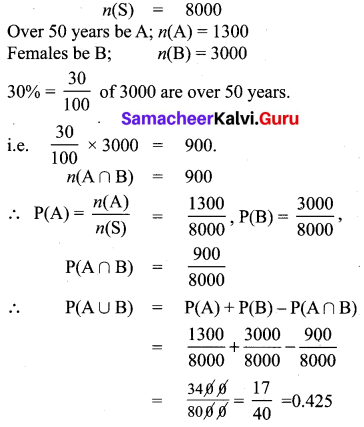Class 10 Maths Chapter 8.4 Solutions Question 12.
A coin is tossed thrice. Find the probability of getting exactly two heads or at least one tail or two consecutive heads.
Solution:
Three coins tossed simultaneously.
S = { HHH, TTT, HHT, TTH, HTH, THT, HTT, THH}
n(S) = 8
Happening of getting exactly two heads be A.
A= {HHT, HTH, THH}
n(A) = 3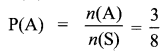Event of getting at least one tail be B.
∴ B = {TTT, HHT, TTH, HTH, THT, HTT, THH}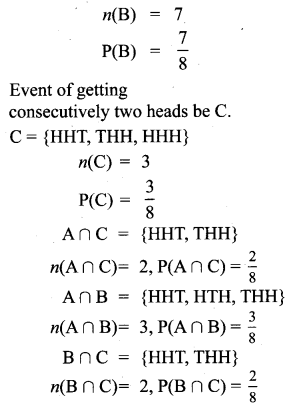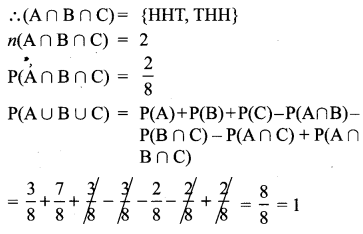10th Samacheer Maths Question 13.
If A, B, C are any three events such that probability of B is twice as that of probability of A and probability of C is thrice as that of probability of A and if P(A∩B) = $$\frac{1}{6}$$, P(B∩C) = $$\frac{1}{4}$$, P(A∩C) = $$\frac{1}{8}$$, $$\mathbf{P}(\mathbf{A} \cup \mathbf{B} \cup \mathbf{C})=\frac{9}{10}, \mathbf{P}(\mathbf{A} \cap \mathbf{B} \cap \mathbf{C})=\frac{1}{15}$$, then find P(A), P(B) and P(C)?
Solution:
P(B) = 2P(A)
P(C) = 3P(A)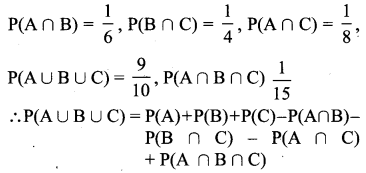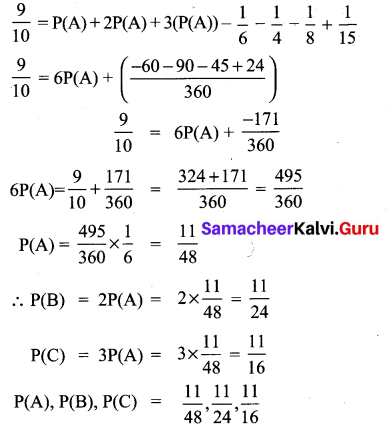Samacheer Kalvi Guru 10th Maths Book Solutions Question 14.
In a class of 35, students are numbered from 1 to 35. The ratio of boys to girls is 4:3. The roll numbers of students begin with boys and end with girls. Find the probability that a student selected is either a boy with a prime roll number or a girl with a composite roll number or an even roll number.
Solution:
n(S) = 35